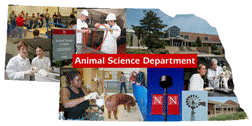## Animal Science Department## Faculty Papers and Publications in Animal Science

#### Date of this Version

March 1999

Published in Journal of Dairy Science Vol. 82, No. 7, 1999.

#### Abstract

A total of 138,869 lactation milk yields (305 d, milked twice daily, mature equivalent) from the first three parities of 68,063 New York Holstein cows were used to estimate variance components that were due to additive direct genetic effects, cow permanent environmental effects (cow within sire for sire model), sire by herd interaction effects, and cytoplasmic line effects. The original data were assigned to 10 random samples, which were each analyzed using an animal model and a sire model. From each sample of original data, 20 other samples were analyzed with levels assigned randomly to cytoplasmic and interaction effects (data with randomly simulated levels). Ten of those samples were analyzed with an animal model and 10 with a sire model. The models also included fixed effects of herd-year-seasons. For the animal model and sire model, average fractions of phenotypic variance and average standard errors were, respectively, for additive direct genetic effects 0.300 (0.029) and 0.228 (0.040) for original data and 0.325 (0.025) and 0.262 (0.039) for data with randomly simulated levels. For cow permanent environmental effects the respective averages were 0.242 (0.024) and 0.444 (0.014) for original data and 0.235 (0.025) and 0.492 (0.016) for data with randomly simulated levels. The averages for sire by herd interaction effects were 0.015 (0.008) and 0.018 (0.007) for original data and 0.003 (0.007) and 0.004 (0.009) for data with randomly simulated levels. For cytoplasmic line effects, the respective averages were 0.011 (0.007) and 0.043 (0.008) for original data and 0.003 (0.006) and 0.003 (0.007) for data with randomly simulated levels. The differences between estimates of variance components for original data and data with randomly simulated levels suggest that estimates of fractions of total variance caused by sire by herd interaction and cytoplasmic effects estimated with REML may be biased upward by 0.003 to 0.004.

COinS